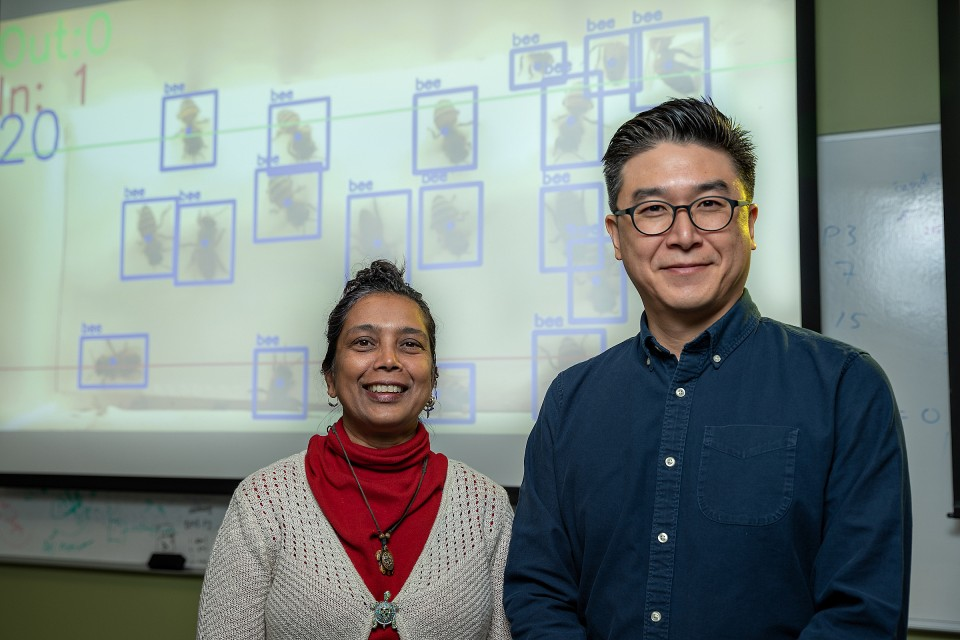## Computer Science Faculty Publications and Presentations

Article

5-6-2019

#### Abstract

We analyze the complexity of building linear assemblies, sets of linear assemblies, and O(1)-scale general shapes in the staged tile assembly model. For systems with at most b bins and t tile types, we prove that the minimum number of stages to uniquely assemble a 1 n line is (logt n + logb n t + 1). Generalizing to O(1) n lines, we prove the minimum number of stages is O( log n tb t log t b2 + log log b log t ) and

( log n tb t log t b2 ). Next, we consider assembling sets of lines and general shapes using t = O(1) tile types. We prove that the minimum number of stages needed to assemble a set of k lines of size at most O(1) n is O( k log n b2 + k p log n b + log log n) and ( k log n b2 ). In the case that b = O( p k), the minimum number of stages is (log n). The upper bound in this special case is then used to assemble \hefty" shapes of at least logarithmic edge-length-to- edge-count ratio at O(1)-scale using O( p k) bins and optimal O(log n) stages.

© 2019, Springer Nature B.V. Original published version available at https://doi.org/10.1007/s11047-019-09740-y

527

548

#### Publication Title

Natural Computing

#### DOI

10.1007/s11047-019-09740-y

COinS